# ISEE Lower Level Quantitative : How to find the area of a parallelogram

## Example Questions

← Previous 1 3 4 5 6 7 8 9 19 20

### Example Question #42 : Quadrilaterals

What is the area of a parallelogram with a height ofinches and a base ofinches?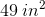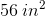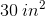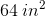Explanation:

The formula for finding the area of a parallelogram is the same as that of finding the area of a rectangle: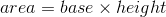In this case, the area equalsinches timesinches, or.

### Example Question #1 : How To Find The Area Of A Parallelogram

Ted drew the parallelogram shown below.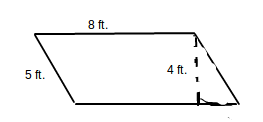What is the area of the parallelogram?

40 square feet

20 square feet

26 square feet

160 square feet

32 square feet

32 square feet

Explanation:

The area of a parallelogram can be found by multiplying the base times the height.  The height is the perpendicular distance between two bases.  In the above parallelogram, the base is 8 and its height is 4, giving the equation 8 x 4 = A.  The area is 32 square feet.

### Example Question #42 : Quadrilaterals

Wilhelmina drew the parallelogram below.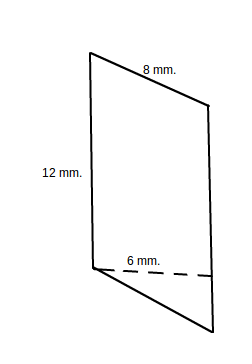What is the area of the parallelogram?

72 square millimeters

40 square millimeters

80 square millimeters

42 square millimeters

96 square millimeters

72 square millimeters

Explanation:

The area of a parallelogram can be found by multiplying the base times the height.  The height is the perpendicular distance between two bases.  In the above parallelogram, the base is 12 and its height is 6, giving the equation 12 x 6 = A.  The area is 72 square millimeters.

### Example Question #43 : Quadrilaterals

Find the area of the following parallelogram.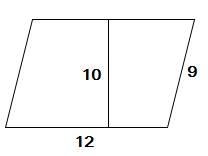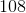Explanation:

In order to find the area of a parallelogram we use the equation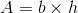In this case our values are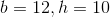So, our area is: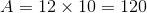### Example Question #2 : How To Find The Area Of A Parallelogram

Find the area of the following parallelogram.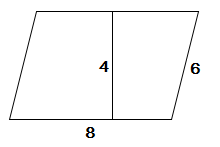Explanation:

The equation for the area of a parallelogram is.

In this case, our values are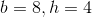so, the area is: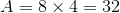### Example Question #1 : Find Area By Partitioning Shapes Into Equal Parts: Ccss.Math.Content.3.G.A.2

What area of the rectangle is filled in?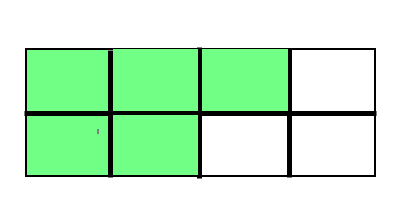Explanation:

Area is the amount of space within the perimeter of a two-dimensional shape. The rectangle is split intopieces andof those pieces are filled in.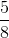of the area of this rectangle is filled in.

### Example Question #2 : Find Area By Partitioning Shapes Into Equal Parts: Ccss.Math.Content.3.G.A.2

What area of the rectangle is filled in?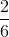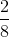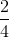Explanation:

Area is the amount of space within the perimeter of a two-dimensional shape. The rectangle is split intopieces andof those pieces are filled in.of the area of this rectangle is filled in.

### Example Question #3 : Find Area By Partitioning Shapes Into Equal Parts: Ccss.Math.Content.3.G.A.2

What area of the rectangle is filled in?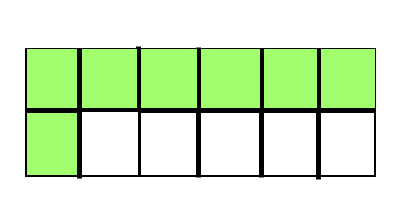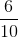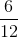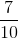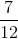Explanation:

Area is the amount of space within the perimeter of a two-dimensional shape. The rectangle is split intopieces andof those pieces are filled in.of the area of this rectangle is filled in.

### Example Question #62 : Rectangles

What area of the rectangle is filled in?Explanation:

Area is the amount of space within the perimeter of a two-dimensional shape. The rectangle is split intopieces andof those pieces is filled in.of the area of this rectangle is filled in.

### Example Question #5 : Find Area By Partitioning Shapes Into Equal Parts: Ccss.Math.Content.3.G.A.2

What area of the rectangle is filled in?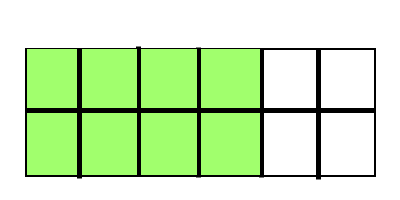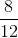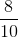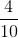Area is the amount of space within the perimeter of a two-dimensional shape. The rectangle is split intopieces andof those pieces are filled in.of the area of this rectangle is filled in.